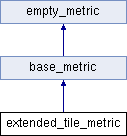extended_tile_metric Class Reference

`#include <extended_tile_metric.h>`

Inheritance diagram for extended_tile_metric:## Public Types

enum  { TYPE = constants::ExtendedTile, LATEST_VERSION = 3 }Public Types inherited from base_metric
enum  {
LANE_BIT_COUNT = 6, TILE_BIT_COUNT = 26, CYCLE_BIT_COUNT = 16, READ_BIT_COUNT = 16,
RESERVED_BIT_COUNT = 16, READ_BIT_SHIFT = RESERVED_BIT_COUNT, CYCLE_BIT_SHIFT = RESERVED_BIT_COUNT, TILE_BIT_SHIFT = CYCLE_BIT_SHIFT+CYCLE_BIT_COUNT,
LANE_BIT_SHIFT = TILE_BIT_SHIFT+TILE_BIT_COUNT
}

typedef ::uint64_t ulong_t

typedef ulong_t id_t

typedef ::uint16_t ushort_t

typedef ::uint32_t uint_t

typedef ::uint8_t lane_t

typedef ::uint32_t tile_t

typedef constants::base_tile_t base_tPublic Types inherited from empty_metric
typedef ::uint32_t id_t

typedef ::uint32_t uint_t

typedef constants::base_run_t base_t

## Public Member Functions

extended_tile_metric ()

extended_tile_metric (const uint_t lane, const uint_t tile, const float occupied, const point2d &upper_left=point2d())

void set (const uint_t lane, const uint_t tile, const float occupied, const point2d &upper_left=point2d())

float cluster_count_occupied () const

float cluster_count_occupied_k () const

float percent_occupied () const

const point2dupper_left () const

void set_cluster_count_k (const float cluster_count_k)Public Member Functions inherited from base_metric
base_metric (const uint_t lane=0, const uint_t tile=0)

void set_base (const uint_t lane, const uint_t tile)

template<class BaseMetric >
void set_base (const BaseMetric &base)

id_t id () const

id_t tile_hash () const

uint_t lane () const

uint_t tile () const

uint_t number (const illumina::interop::constants::tile_naming_method) const

uint_t section (const illumina::interop::constants::tile_naming_method method) const

uint_t surface (const illumina::interop::constants::tile_naming_method method) const

uint_t swath (const illumina::interop::constants::tile_naming_method method) const

uint_t phyiscalLocationIndex (const illumina::interop::constants::tile_naming_method method, const uint_t section_per_lane, const uint_t tile_count, const uint_t swath_count, const bool all_surfaces) const

uint_t phyiscalLocationColumn (const illumina::interop::constants::tile_naming_method method, const uint_t swath_count, const bool all_surfaces) const

uint_t phyiscalLocationRow (const illumina::interop::constants::tile_naming_method method, const uint_t section_per_lane, const uint_t tile_count) const

size_t physical_location_index (const illumina::interop::constants::tile_naming_method method, const uint_t section_per_lane, const uint_t tile_count, const uint_t swath_count, const bool all_surfaces) const

uint_t physical_location_column (const illumina::interop::constants::tile_naming_method method, const uint_t swath_count, const bool all_surfaces) const

uint_t physical_location_row (const illumina::interop::constants::tile_naming_method method, const uint_t section_per_lane, const uint_t tile_count) const

bool operator< (const base_metric &metric2) constPublic Member Functions inherited from empty_metric
template<class BaseMetric >
void set_base (const BaseMetric &)

void set_base (const uint_t, const uint_t)

bool operator< (const empty_metric &) const

## Static Public Member Functions

static const char * prefix ()Static Public Member Functions inherited from base_metric
static id_t create_id (const id_t lane, const id_t tile, const id_t=0)

static id_t lane_from_id (const id_t id)

static id_t tile_hash_from_id (const id_t id)

static id_t tile_from_id (const id_t id)

static const char * suffix ()Static Public Member Functions inherited from empty_metric
static const char * suffix ()

static id_t create_id (const id_t, const id_t, const id_t=0)

## Friends

template<class MetricType , int Version>
struct io::generic_layout

## Detailed Description

Extended tile metric

The extended tile metric reports tile metrics such as the occupancy aka number of empty wells on a tile.

Note
Supported versions: 2 and 3
Test:

Confirm version 1 of the metric can be written to and read from a stream

Confirm version 1 of the metric matches known binary file

## Member Enumeration Documentation

 anonymous enum
Enumerator
TYPE

Unique type code for metric

LATEST_VERSION

## Constructor & Destructor Documentation

 extended_tile_metric ( )
inline

Constructor

 extended_tile_metric ( const header_type & )
inline

Constructor

 extended_tile_metric ( const uint_t lane, const uint_t tile, const float occupied, const point2d & upper_left = `point2d()` )
inline

Constructor

Parameters
 lane lane number tile tile number occupied number of occupied wells upper_left coordinate of upper left fiducial

## Member Function Documentation

 static const char* prefix ( )
inlinestatic

Get the prefix of the InterOp filename

Returns
"ExtendedTile"
 void set ( const uint_t lane, const uint_t tile, const float occupied, const point2d & upper_left = `point2d()` )
inline

Setter

Note
Version 1 and 2
Parameters
 lane lane number tile tile number occupied number of occupied wells upper_left coordinate of upper left fiducial
 void set_cluster_count_k ( const float cluster_count_k )
inline

Set the cluster count in kilo bases

Derived from tile_metric using `populate_percent_occupied` in `run_metrics::finalize_after_load`

Parameters
 cluster_count_k cluster count in kilo bases

## Friends And Related Function Documentation

 friend struct io::generic_layout
friend

The documentation for this class was generated from the following file: